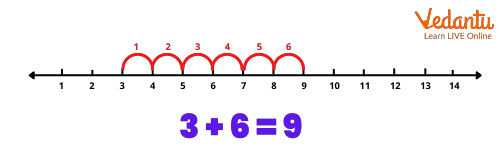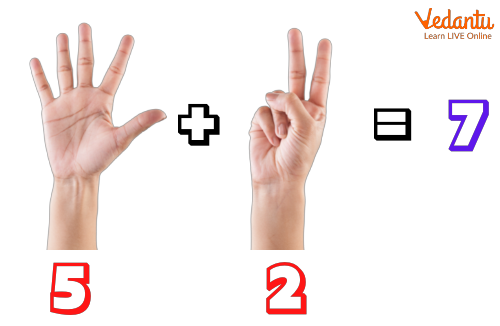Courses
Courses for Kids
Free study material
Free LIVE classes
More

# Addition: Meaning, Use and Symbol## Learn the Meaning of Addition with Examples

Let us imagine that you have five oranges and three apples. Both oranges and apples are fruits. So, if I ask you to tell me the total number of fruits you have, what will you do? You will count the number of oranges and the number of apples that you have and make the total. This method is called addition.

Addition is one of the four basic operations of Mathematics, with the other three being subtraction, multiplication, and division. The addition means finding the total or sum by combining two or more numbers. Let’s learn what addition means with the help of another example below.From the above image, we can see one red ball and one blue ball. If we add them, we will get a total of two balls. In numeral form, it is represented as: 1 + 1 = 2

And in words, it is written as one plus one equals two.

The symbol used to represent addition is (+). The addition symbol joins two or more numbers and gives the complete expression.

For example, (2 + 4 = 6).

Here, number 2 is added to another number 4 to obtain the answer 6.

The addition symbol (+) is also known as the ‘plus sign’ or ‘addition sign’ and consists of one horizontal line and one vertical line.

The addition formula is expressed as:

Note: While adding numbers or objects, we use two symbols namely addition symbol (+), and the other is equal to sign (=).

Also, it should be noted that there can be multiple addends in addition. For example:

2 + 4 + 6 = 12

Here, 2, 4, and 6 are addends and 12 is the sum.

We can add two or more numbers using the number line. Let us understand with an example:

Example:

Add 3 + 6 using the number line.

Here, we will first mark the number 3 on the number line.

Now, as we have to add 6 to 3, we will move six steps to the right of 3.

This gives us the answer 9. Hence, 3 + 6 = 9The addition of small numbers can also be done using fingers. Check the example below to find how to add small numbers using fingers.

Add 5 and 2 using the fingers.

Following are the steps to add 5 and 2 using fingers:

• Step 1: Hold up 5 on one hand.

• Step 2: Hold up 2 on the other hand.

• Step 3: Count all your fingers.

The result obtained is 7.When the addition of the digits is less than or equal to 9 in each column, then it is termed as addition without regrouping.

Let us understand addition without regrouping with an example:

Example: 22334 + 42562

• Step 1 : Start adding the digits in the one’s place (4 + 2 = 6).

• Step 2: Move to the digits is 10’s place (3 + 6 = 9)

• Step 3: Move to the digits in 100’s place (3 + 5 = 8)

• Step 4: Move to the digits in 1000’s place (2 + 2 = 4)

• Step 5: Finally, add the digits in 10,000’s place (2 + 4= 6)

Therefore, 22334 + 42562 = 64894

Ten Thousands Thousands Hundreds Tens Ones

2 2 3 3 4

+ 4 2 5 6 2

-----------------------------------------------------------------------

6 4 8 9 4

…………………………………………………………………………………..

Note: In addition to regrouping, we simply add the digits in each place value column and read the respective total or sum together to get the answer.

If the addition of the digits is more than 9 in each column, it is termed as addition with regrouping. In addition with regrouping, we start adding from one’s digit. If the sum of one's digit is more than 9, then we will write one digit of the sum under the one's column and carry forward its tens digit in the ten’s column.

Let us understand addition with regrouping with an example:

• Step 1: Start adding the digits in one column. Here, the sum of 5 + 7 is 12. The tens digit of the sum i.e. 1 will be carried to the ten’s column.

• Step 2: Start adding the digits in the tens column along with carryover 1. Now, the sum of the ten's column will be 11 (7 + 3 + 1). The tens digit of the sum i.e. 1 will be carried to the hundred’s column.

• Step 3: Start adding the digits in the hundreds column along with carryover 1. Now, the sum of the hundred's column will be 14 (4 + 9 + 1). The tens digit of the sum i.e. 1 will be carried to the thousands column.

• Step 4: Start adding the digits in the thousands column along with carryover 1. Now, the sum of the thousand’s column will be 14 (5 + 8 + 1). The tens digit of the sum i.e. 1 will be carried to the ten thousand’s column.

• Step 5: Start adding the digits in the thousands column along with carryover 1. Now, the sum of ten thousand columns will be 4 (2 + 1 + 1).

Ten Thousand Thousand Hundreds Tens Ones

(1) (1) (1) (1)

2 5 4 3 5

+ 1 8 9 7 7

-----------------------------------------------------------------------

4 4 4 1 2

…………………………………………………………………………………..

Therefore, the sum of 25435 + 18977 = 44412

## Did You Know?

• Adding the digit 0 to any number obtains the result as the number itself.

• When you add 1 to any number, the result will be the successor of that number.

• When you add a set of numbers, the result will be the same no matter in which order you add the numbers. For example, 5 + 3 + 4 = 12 and 4 + 3 + 5 = 12. This is also known as the associative property of addition.

• The words like ‘altogether’, ‘in all’, ‘total’, ‘put together’ or ‘sum’ gives you a hint that you have to add the given numbers.

## Conclusion

In short, addition means combining two or more numbers or objects together to get a new object or number. Hope you can now solve the maximum problems based on addition. To know more about the term addition in Maths, visit our website.

Last updated date: 25th Sep 2023
Total views: 135.3k
Views today: 1.35k

## FAQs on Addition: Meaning, Use and Symbol

1. What are the four different properties of addition?

The four different properties of addition include the following:

• Closure Property: This property states that when we add two or more whole numbers, the result obtained is always a whole number.

• Commutative Property:  This property states that when we add two or more whole numbers, the result obtained is the same regardless of the order of their addends.

• Associative Property: This property states that when we add three or more numbers, the result obtained is the same regardless of the order of their addend.

• Identity Elements of Addition: When we add 0 to any number, the result obtained is the same as the original number. Adding 0 to any number does not change the value of the original number.

2. Give an example of addition as an increase?

The addition also means to make it bigger. For example,

• Harry has a house. If he puts one new room in the house. This new room is called addition.

• Tony is making juice. To make the juice taste better, he puts some sugar in the juice. This means Tony added sugar to the juice. Sugar is a sweet additive or addition to the juice.